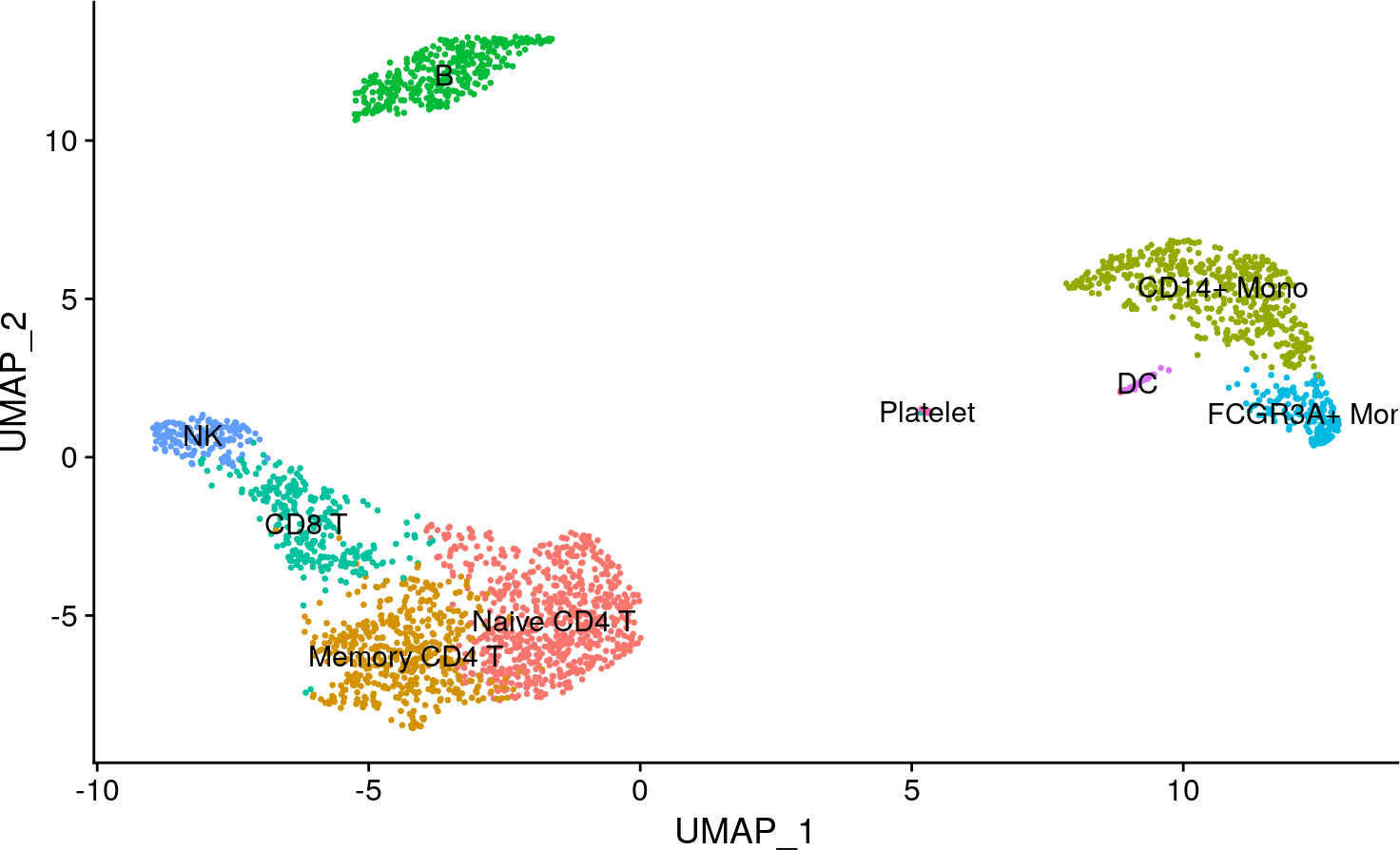# 1 Introduction

DoRothEA is a comprehensive resource containing a curated collection of transcription factors (TFs) and its transcriptional targets. The set of genes regulated by a specific transcription factor is known as regulon. DoRothEA’s regulons were gathered from different types of evidence. Each TF-target interaction is defined by a confidence level based on the number of supporting evidence. The confidence levels ranges from A (highest confidence) to E (lowest confidence) (Garcia-Alonso et al. 2019).

DoRothEA regulons are usually coupled with the statistical method VIPER (Alvarez et al. 2016). In this context, TF activities are computed based on the mRNA expression levels of its targets. We therefore can consider TF activity as a proxy of a given transcriptional state (Dugourd and Saez-Rodriguez 2019).

Holland et al. (2020) evaluated the performance of DoRothEA in combination with VIPER when applied to scRNA-seq data. We showed that, in spite of the current limitations of scRNA-seq technologies, their approach can provide meaningful results in this context. Indeed, this vignette shows an example on how to apply DoRothEA regulons coupled with VIPER in a well known single-cell dataset.

# 2 Installation

First of all, you need a current version of R (http://www.r-project.org). DoRothEA is a freely available annotation package deposited on http://bioconductor.org/ and https://github.com/saezlab/dorothea.

You can install it by running the following commands on an R console:

``````if (!requireNamespace("BiocManager", quietly = TRUE))
install.packages("BiocManager")

BiocManager::install("dorothea")``````

We also load here the packages required to run this script.

``````## We load the required packages
library(dorothea)
library(dplyr)
library(Seurat)
library(tibble)
library(pheatmap)
library(tidyr)
library(viper)``````

# 3 Example of usage

In the following paragraphs, we provide examples describing how to run VIPER on DoRothEA regulons in a scRNA-seq dataset. In particular, we use the Seurat toolkit for single cell genomics (Stuart et al. 2019). For the sake of simplicity, we follow the example provided in the following Seurat vignette:

https://satijalab.org/seurat/v3.1/pbmc3k_tutorial.html

The dataset contains 2700 Peripheral Blood Mononuclear Cells (PBMC) that were sequenced on the Illumina NextSeq 500. This dataset is freely available in 10X Genomics:

https://cf.10xgenomics.com/samples/cell/pbmc3k/pbmc3k_filtered_gene_bc_matrices.tar.gz

``````## Load the PBMC dataset
pbmc.data <- Read10X(data.dir = "filtered_gene_bc_matrices/hg19/")

## Initialize the Seurat object with the raw (non-normalized data).
pbmc <- CreateSeuratObject(counts = pbmc.data, project = "pbmc3k",
min.cells = 3, min.features = 200)``````

## 3.1 Pre-processing, normalization and identification of highly variable features

We follow the standard pre-processing steps as described in the aforementioned Seurat vignette before going deeper into the data analysis. These steps carry out the selection and filtration of cells based on quality control metrics, the data normalization and scaling, and the detection of highly variable features (see https://satijalab.org/seurat/v3.1/pbmc3k_tutorial.html).

``````## Identification of mithocondrial genes
pbmc[["percent.mt"]] <- PercentageFeatureSet(pbmc, pattern = "^MT-")

## Filtering cells following standard QC criteria.
pbmc <- subset(pbmc, subset = nFeature_RNA > 200 & nFeature_RNA < 2500 &
percent.mt < 5)

## Normalizing the data
pbmc <- NormalizeData(pbmc, normalization.method = "LogNormalize",
scale.factor = 10000)

pbmc <- NormalizeData(pbmc)

## Identify the 2000 most highly variable genes
pbmc <- FindVariableFeatures(pbmc, selection.method = "vst", nfeatures = 2000)

## In addition we scale the data
all.genes <- rownames(pbmc)
pbmc <- ScaleData(pbmc, features = all.genes)``````

## 3.2 Clustering cells

One of the most relevant steps in scRNA-seq data analysis is clustering. Cells are grouped based on the similarity of their transcriptomic profiles. We first apply the Seurat v3 classical approach as described in their aforementioned vignette. We visualize the cell clusters using UMAP:

``````pbmc <- RunPCA(pbmc, features = VariableFeatures(object = pbmc),
verbose = FALSE)
pbmc <- FindNeighbors(pbmc, dims = 1:10, verbose = FALSE)
pbmc <- FindClusters(pbmc, resolution = 0.5, verbose = FALSE)
pbmc <- RunUMAP(pbmc, dims = 1:10, umap.method = "uwot", metric = "cosine")

pbmc.markers <- FindAllMarkers(pbmc, only.pos = TRUE, min.pct = 0.25,
logfc.threshold = 0.25, verbose = FALSE)

## Assigning cell type identity to clusters
new.cluster.ids <- c("Naive CD4 T", "Memory CD4 T", "CD14+ Mono", "B", "CD8 T",
"FCGR3A+ Mono", "NK", "DC", "Platelet")
names(new.cluster.ids) <- levels(pbmc)
pbmc <- RenameIdents(pbmc, new.cluster.ids)``````
``DimPlot(pbmc, reduction = "umap", label = TRUE, pt.size = 0.5) + NoLegend()``## 3.3 Clustering cells with TF activity

Holland et al. (2020) showed that clustering the cells based on their TF activity profiles can also be very interesting. Indeed, clustering cells using TF activity computed with VIPER and DoRothEA performs better than using the expression level of the same TFs. In addition, it brings complementary information to the clusters based on transcriptomics profiles.

Here, we first run VIPER on DoRothEA’s regulons to obtain TFs activity, by using the wrapper function `run_viper()`. This function can deal with different input types such as matrix, dataframe, ExpressionSet or even Seurat objects. In case of a seurat object the function returns the same seurat object with an additonal assay called `dorothea` containing the TF activities in the slot `data`.

``````## We read Dorothea Regulons for Human:
dorothea_regulon_human <- get(data("dorothea_hs", package = "dorothea"))

## We obtain the regulons based on interactions with confidence level A, B and C
regulon <- dorothea_regulon_human %>%
dplyr::filter(confidence %in% c("A","B","C"))

## We compute Viper Scores
pbmc <- run_viper(pbmc, regulon,
options = list(method = "scale", minsize = 4,
eset.filter = FALSE, cores = 1,
verbose = FALSE))``````

We then apply Seurat to cluster the cells following the same protocol than above but using TF activity scores.

``````## We compute the Nearest Neighbours to perform cluster
DefaultAssay(object = pbmc) <- "dorothea"
pbmc <- ScaleData(pbmc)
pbmc <- RunPCA(pbmc, features = rownames(pbmc), verbose = FALSE)
pbmc <- FindNeighbors(pbmc, dims = 1:10, verbose = FALSE)
pbmc <- FindClusters(pbmc, resolution = 0.5, verbose = FALSE)

pbmc <- RunUMAP(pbmc, dims = 1:10, umap.method = "uwot", metric = "cosine")

pbmc.markers <- FindAllMarkers(pbmc, only.pos = TRUE, min.pct = 0.25,
logfc.threshold = 0.25, verbose = FALSE)

## Assigning cell type identity to clusters
new.cluster.ids <- c("Naive CD4 T", "Memory CD4 T", "CD14+ Mono", "B", "CD8 T",
"FCGR3A+ Mono", "NK", "DC", "Platelet")
names(new.cluster.ids) <- levels(pbmc)
pbmc <- RenameIdents(pbmc, new.cluster.ids)``````
``````## Warning: Cannot find identity NA

## Warning: Cannot find identity NA``````
``DimPlot(pbmc, reduction = "umap", label = TRUE, pt.size = 0.5) + NoLegend()``